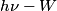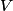## Solution to 1986 Problem 33

 The correct answer is (D). The quantity$h \nu - W$ is the kinetic energy of the electron after it is ejected from the metal. This is the electron's kinetic energy budget that it can use to try to reach the collector. Assuming$V$ is negative, this kinetic energy energy is converted to potential energy as the electron travels towards the photocathode. Whether or not the electron has any kinetic energy left by the time it reaches the photocathode determines whether or not there will be a measured current.